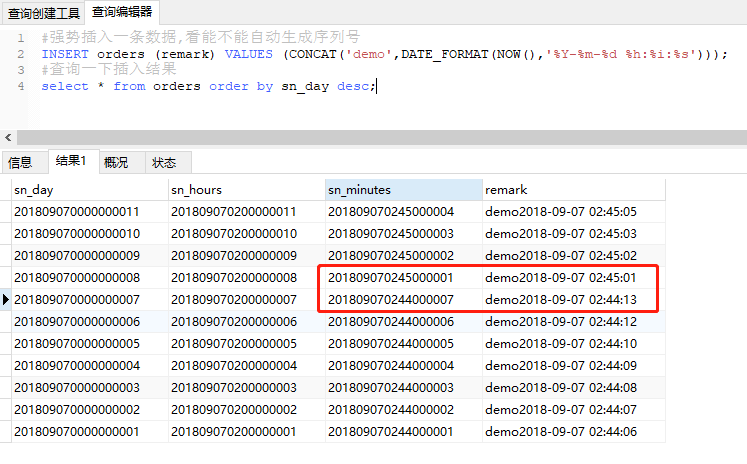# MySQL 根据触发器编写自定义流水号

1.首先我们创建一张订单表

sn_day,记录按天生成的序列号; sn_hours,记录按小时生成的序列号; sn_minutes 表示按分生成的序列号;

CREATE TABLE orders (
sn_day DECIMAL (32) PRIMARY KEY,
sn_hours DECIMAL (32),
sn_minutes DECIMAL (32),
remark VARCHAR (100)
);

2.我们在这订单表新增数据时,自动生成序列号.而不是我们主动去插入这个序列号.那么就需要用到触发器.顾名思义,触发嘛.

CREATE TRIGGER sn_by_day BEFORE INSERT ON orders FOR EACH ROW
BEGIN
DECLARE
n INT;

SELECT
IFNULL(max(RIGHT(sn_day, 10)), 0) INTO n
FROM
orders
WHERE
mid(sn_day, 1, 8) = DATE_FORMAT(NOW(), '%Y%m%d');

SET NEW.sn_day = concat(
DATE_FORMAT(NOW(), '%Y%m%d'),
RIGHT (10000000001 + n, 10)
);

END;

create trigger sn_by_day 创建一个触发器,名称叫 sn_by_day

before insert 在插入前

on orders 在订单表上

1.先定义一个变量,叫n
2.在订单表中查询 sn_day 字段值的1-8位等于当前日期的数据
3.在这个数据中,取后10位最大的,如果没有,则为0
4.将这个查询出来的值赋给变量n
5.将变量n加上10000000001,当然,要加上多少,你可以自己定.主要是为了蹭长度
6.在当前日期将这个值呢拼接上,然后就生成了一个以当前日期开头的增长序列值
7.将这个增长序列值存到 sn_day 字段中.

#强势插入一条数据,看能不能自动生成序列号
INSERT orders (remark) VALUES (CONCAT('demo',DATE_FORMAT(NOW(),'%Y-%m-%d %h:%i:%s')));CREATE TRIGGER sn_by_hours BEFORE INSERT ON orders FOR EACH ROW
BEGIN
DECLARE
n INT;

SELECT
IFNULL(max(RIGHT(sn_hours, 8)), 0) INTO n
FROM
orders
WHERE
mid(sn_hours, 1, 10) = FROM_UNIXTIME(
UNIX_TIMESTAMP(NOW(6)),
'%Y%m%d%h'
);

SET NEW.sn_hours = concat(
FROM_UNIXTIME(
UNIX_TIMESTAMP(NOW(6)),
'%Y%m%d%h'
),
RIGHT (100000001 + n, 8)
);

END;

CREATE TRIGGER sn_by_minutes BEFORE INSERT ON orders FOR EACH ROW
BEGIN
DECLARE
n INT;

SELECT
IFNULL(max(RIGHT(sn_minutes, 6)), 0) INTO n
FROM
orders
WHERE
mid(sn_minutes, 1, 12) = FROM_UNIXTIME(
UNIX_TIMESTAMP(NOW(6)),
'%Y%m%d%h%i'
);

SET NEW.sn_minutes = concat(
FROM_UNIXTIME(
UNIX_TIMESTAMP(NOW(6)),
'%Y%m%d%h%i'
),
RIGHT (1000001 + n, 6)
);

END;